Tanishq Gautam — Published On January 20, 2021 and Last Modified On January 20th, 2021

## Overview

• Learning about the state of the art model that is Transformers.
• Understand how we can implement Transformers on the already seen image captioning problem using Tensorflow
• Comparing the results of Transformers vs attention models.

## Introduction

We have seen that Attention mechanisms (in the previous article) have become an integral part of compelling sequence modeling and transduction models in various tasks (such as image captioning), allowing modeling of dependencies without regard to their distance in the input or output sequences.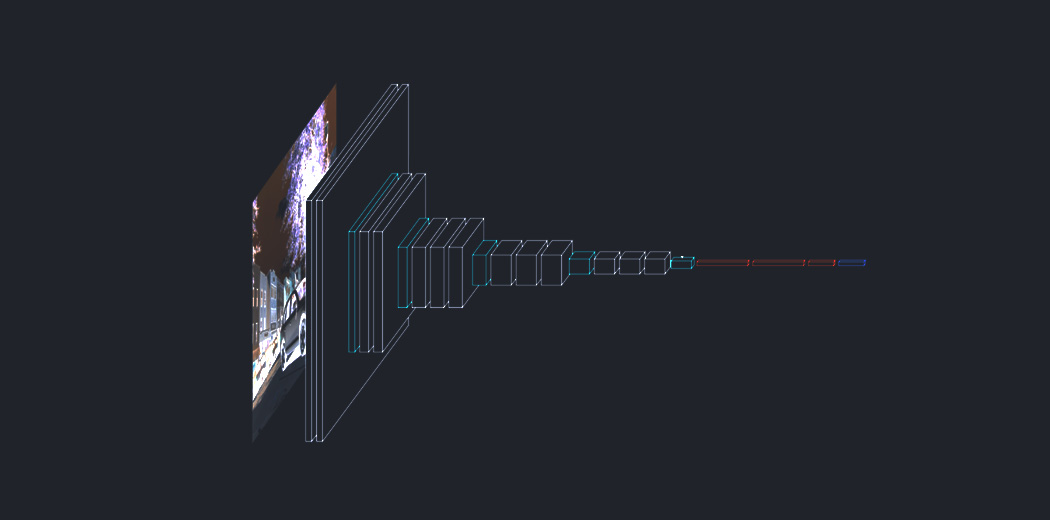The Transformer, a model architecture eschewing recurrence and instead relying entirely on an attention mechanism to draw global dependencies between input and output. The Transformer architecture allows for significantly more parallelization and can reach new state of the art results in translation quality.

In this article, let’s see how you can implement the Attention Mechanism for Caption Generation with Transformers using TensorFlow.

Prerequisites before you get started:-

1. Transformer Architecture
2. Implementation of Attention Mechanism for Caption Generation with Transformers using Tensorflow
1. Importing required libraries
3. Model definition
4. Positional Encoding
6. Encoder-Decoder Layer
7. Transformer
8. Model Hyperparameters
9. Model training
10. BLEU Evaluation
11. Comparison
3. What’s Next?
4. End Notes

## Transformers Architecture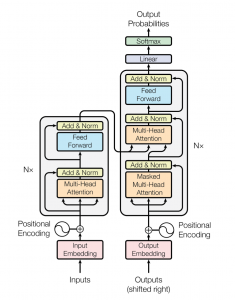The transformer network employs an encoder-decoder architecture similar to that of an RNN. The main difference is that transformers can receive the input sentence/sequence in parallel, i.e, there is no time step associated with the input, and all the words in the sentence can be passed simultaneously.

Let’s begin with understanding the input to the transformer.

Consider an English to German translation. We feed the entire English sentence to the input embedding. An input embedding layer can be thought of as a point in space where similar words in meaning are physically closer to each other, i.e, each word maps to a vector with continuous values to represent that word.

Now a problem with this is that the same word in different sentences can have different meanings this is where position encoding enters. Since transformers contain no recurrence and no convolution, in order for the model to make use of the order of the sequence, it must make use of some information about the relative or absolute position of words in a sequence. The idea is to use fixed or learned weights that encode information related to a specific position of a token in a sentence.

Similarly, the target German word is fed into the output embedding and its positional encoding vector is passed into the decoder block.

The encoder block has two sub-layers. The first is a multi-head self-attention mechanism, and the second is a simple, position-wise fully connected feed-forward network. For every word, we can have an attention vector generated that captures contextual relationships between words in a sentence. Multi-headed attention in the encoder applies a specific attention mechanism called self-attention. Self-attention allows the models to associate each word in the input, to other words.

In addition to the two sub-layers in each encoder layer, the decoder inserts a third sub-layer, which performs multi-head attention over the output of the encoder stack. Similar to the encoder, we employ residual connections around each of the sub-layers, followed by layer normalization. The attention vectors of the german words and the attention vectors of English sentences from the encoder are passed into second multi head attention.

This attention block will determine how related each word vector is with respect to each other. This is where the English to german word mapping takes place. The decoder is capped off with a linear layer that acts as a classifier, and a softmax to get the word probabilities.

Now that you have got a basic overview of how transformers work let’s see how we can implement it for the image captioning task using Tensorflow and compare our results with other methods.

## Implementation of Attention Mechanism for Caption Generation with Transformers using TensorFlow

You can find the entire source code on my Github profile.

#### Step 1:- Import the required libraries

Here we will be making use of Tensorflow for creating our model and training it. The majority of the code credit goes to TensorFlow tutorials. You can make use of Google Colab or Kaggle notebooks if you want a GPU to train it.

```import string
import numpy as np
import pandas as pd
from numpy import array
from PIL import Image
import pickle

import matplotlib.pyplot as plt
import sys, time, os, warnings
warnings.filterwarnings("ignore")
import re

import keras
import tensorflow as tf
from tqdm import tqdm
from nltk.translate.bleu_score import sentence_bleu

from keras.utils import to_categorical
from keras.utils import plot_model
from keras.models import Model
from keras.layers import Input
from keras.layers import Dense, BatchNormalization
from keras.layers import LSTM
from keras.layers import Embedding
from keras.layers import Dropout
from keras.callbacks import ModelCheckpoint
from keras.preprocessing.text import Tokenizer

from sklearn.utils import shuffle
from sklearn.model_selection import train_test_split```

Define our image and caption path and check how many total images are present in the dataset.

```image_path = "/content/gdrive/My Drive/FLICKR8K/Flicker8k_Dataset"
dir_Flickr_text = "/content/gdrive/My Drive/FLICKR8K/Flickr8k_text/Flickr8k.token.txt"
jpgs = os.listdir(image_path)

print("Total Images in Dataset = {}".format(len(jpgs)))```

Output: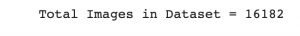We create a dataframe to store the image id and captions for ease of use.

```file = open(dir_Flickr_text,'r')
file.close()

datatxt = []
for line in text.split('\n'):
col = line.split('\t')
if len(col) == 1:
continue
w = col.split("#")
datatxt.append(w + [col.lower()])

data = pd.DataFrame(datatxt,columns=["filename","index","caption"])
data = data.reindex(columns =['index','filename','caption'])
data = data[data.filename != '2258277193_586949ec62.jpg.1']
uni_filenames = np.unique(data.filename.values)

Output: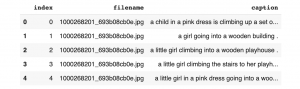Next, let’s visualize a few images and their 5 captions:

```npic = 5
npix = 224
target_size = (npix,npix,3)
count = 1

fig = plt.figure(figsize=(10,20))
for jpgfnm in uni_filenames[10:14]:
filename = image_path + '/' + jpgfnm
captions = list(data["caption"].loc[data["filename"]==jpgfnm].values)
count += 1

plt.axis('off')
ax.plot()
ax.set_xlim(0,1)
ax.set_ylim(0,len(captions))
for i, caption in enumerate(captions):
ax.text(0,i,caption,fontsize=20)
count += 1
plt.show()```

Output: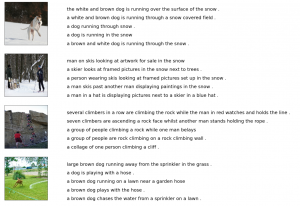Next let’s see what our current vocabulary size is:-

```vocabulary = []
for txt in data.caption.values:
vocabulary.extend(txt.split())
print('Vocabulary Size: %d' % len(set(vocabulary)))```

Output: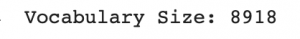Next perform some text cleaning such as removing punctuation, single characters, and numeric values:

```def remove_punctuation(text_original):
text_no_punctuation = text_original.translate(string.punctuation)
return(text_no_punctuation)

def remove_single_character(text):
text_len_more_than1 = ""
for word in text.split():
if len(word) > 1:
text_len_more_than1 += " " + word
return(text_len_more_than1)

def remove_numeric(text):
text_no_numeric = ""
for word in text.split():
isalpha = word.isalpha()
if isalpha:
text_no_numeric += " " + word
return(text_no_numeric)

def text_clean(text_original):
text = remove_punctuation(text_original)
text = remove_single_character(text)
text = remove_numeric(text)
return(text)

for i, caption in enumerate(data.caption.values):
newcaption = text_clean(caption)
data["caption"].iloc[i] = newcaption```

Now let’s see the size of our vocabulary after cleaning-

```clean_vocabulary = []
for txt in data.caption.values:
clean_vocabulary.extend(txt.split())
print('Clean Vocabulary Size: %d' % len(set(clean_vocabulary)))```

Output: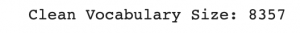Next, we save all the captions and image paths in two lists so that we can load the images at once using the path set. We also add ‘< start >’ and ‘< end >’ tags to every caption so that the model understands the starting and end of each caption.

```PATH = "/content/gdrive/My Drive/FLICKR8K/Flicker8k_Dataset/"
all_captions = []
for caption  in data["caption"].astype(str):
caption = '<start> ' + caption+ ' <end>'
all_captions.append(caption)

all_captions[:10]```

Output: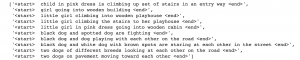```all_img_name_vector = []
for annot in data["filename"]:
full_image_path = PATH + annot
all_img_name_vector.append(full_image_path)

all_img_name_vector[:10]```

Output: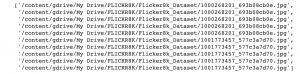Now you can see we have 40455 image paths and captions.

```print(f"len(all_img_name_vector) : {len(all_img_name_vector)}")
print(f"len(all_captions) : {len(all_captions)}")```

Output: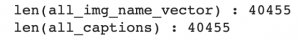We will take only 40000 of each so that we can select batch size properly i.e. 625 batches if batch size= 64. To do this we define a function to limit the dataset to 40000 images and captions.

```def data_limiter(num,total_captions,all_img_name_vector):
train_captions, img_name_vector = shuffle(total_captions,all_img_name_vector,random_state=1)
train_captions = train_captions[:num]
img_name_vector = img_name_vector[:num]
return train_captions,img_name_vector

train_captions,img_name_vector = data_limiter(40000,total_captions,all_img_name_vector)```

#### Step 3:- Model Definition

Let’s define the image feature extraction model using InceptionV3. We must remember that we do not need to classify the images here, we only need to extract an image vector for our images. Hence we remove the softmax layer from the model. We must all preprocess all the images to the same size, i.e, 299×299 before feeding them into the model, and the shape of the output of this layer is 8x8x2048.

```def load_image(image_path):
img = tf.image.decode_jpeg(img, channels=3)
img = tf.image.resize(img, (299, 299))
img = tf.keras.applications.inception_v3.preprocess_input(img)
return img, image_path

image_model = tf.keras.applications.InceptionV3(include_top=False, weights='imagenet')
new_input = image_model.input
hidden_layer = image_model.layers[-1].output
image_features_extract_model = tf.keras.Model(new_input, hidden_layer)```

Next, let’s Map each image name to the function to load the image. We will pre-process each image with InceptionV3 and cache the output to disk and image features are reshaped to 64×2048.

```encode_train = sorted(set(img_name_vector))
image_dataset = tf.data.Dataset.from_tensor_slices(encode_train)

We extract the features and store them in the respective .npy files and then pass those features through the encoder.NPY files store all the information required to reconstruct an array on any computer, which includes dtype and shape information.

```for img, path in tqdm(image_dataset):
batch_features = image_features_extract_model(img)
batch_features = tf.reshape(batch_features,
(batch_features.shape, -1, batch_features.shape))

for bf, p in zip(batch_features, path):
path_of_feature = p.numpy().decode("utf-8")
np.save(path_of_feature, bf.numpy())```

Next, we tokenize the captions and build a vocabulary of all the unique words in the data. We will also limit the vocabulary size to the top 5000 words to save memory. We will replace words not in vocabulary with the token < unk >

```top_k = 5000
tokenizer = tf.keras.preprocessing.text.Tokenizer(num_words=top_k,
oov_token="<unk>",
filters='!"#\$%&()*+.,-/:;=?@[\]^_`{|}~ ')

tokenizer.fit_on_texts(train_captions)
train_seqs = tokenizer.texts_to_sequences(train_captions)

train_seqs = tokenizer.texts_to_sequences(train_captions)

Next, Create training and validation sets using an 80-20 split:

`img_name_train, img_name_val, cap_train, cap_val = train_test_split(img_name_vector,cap_vector, test_size=0.2, random_state=0)`

Next, let’s create a tf.data dataset to use for training our model.

```BATCH_SIZE = 64
BUFFER_SIZE = 1000
num_steps = len(img_name_train) // BATCH_SIZE

def map_func(img_name, cap):
return img_tensor, cap

dataset = tf.data.Dataset.from_tensor_slices((img_name_train, cap_train))
dataset = dataset.map(lambda item1, item2: tf.numpy_function(map_func, [item1, item2], [tf.float32, tf.int32]),num_parallel_calls=tf.data.experimental.AUTOTUNE)
dataset = dataset.shuffle(BUFFER_SIZE).batch(BATCH_SIZE)
dataset = dataset.prefetch(buffer_size=tf.data.experimental.AUTOTUNE)```

#### Step 4:- Positional Encoding

The positional encoding uses sine and cosine functions of different frequencies. For every odd index on the input vector, create a vector using the cos function, for every even index, create a vector using the sin function. Then add those vectors to their corresponding input embeddings which successfully gives the network information on the position of each vector.

```def get_angles(pos, i, d_model):
angle_rates = 1 / np.power(10000, (2 * (i//2)) / np.float32(d_model))
return pos * angle_rates

def positional_encoding_1d(position, d_model):
np.arange(d_model)[np.newaxis, :],
d_model)

return tf.cast(pos_encoding, dtype=tf.float32)

def positional_encoding_2d(row,col,d_model):
assert d_model % 2 == 0
row_pos = np.repeat(np.arange(row),col)[:,np.newaxis]
col_pos = np.repeat(np.expand_dims(np.arange(col),0),row,axis=0).reshape(-1,1)

return tf.cast(pos_encoding, dtype=tf.float32)```

Calculate the attention weights. q, k, v must have matching leading dimensions. k, v must have matching penultimate dimension, i.e.: seq_len_k = seq_len_v. The mask has different shapes depending on its type (padding or look ahead) but it must be broadcastable for addition.

```def create_padding_mask(seq):
seq = tf.cast(tf.math.equal(seq, 0), tf.float32)
return seq[:, tf.newaxis, tf.newaxis, :]  # (batch_size, 1, 1, seq_len)

mask = 1 - tf.linalg.band_part(tf.ones((size, size)), -1, 0)

matmul_qk = tf.matmul(q, k, transpose_b=True)  # (..., seq_len_q, seq_len_k)
dk = tf.cast(tf.shape(k)[-1], tf.float32)
scaled_attention_logits = matmul_qk / tf.math.sqrt(dk)
.

attention_weights = tf.nn.softmax(scaled_attention_logits, axis=-1)
output = tf.matmul(attention_weights, v)  # (..., seq_len_q, depth_v)

return output, attention_weights

self.d_model = d_model
assert d_model % self.num_heads == 0
self.wq = tf.keras.layers.Dense(d_model)
self.wk = tf.keras.layers.Dense(d_model)
self.wv = tf.keras.layers.Dense(d_model)
self.dense = tf.keras.layers.Dense(d_model)

x = tf.reshape(x, (batch_size, -1, self.num_heads, self.depth))
return tf.transpose(x, perm=[0, 2, 1, 3])

def call(self, v, k, q, mask=None):
batch_size = tf.shape(q)
q = self.wq(q)  # (batch_size, seq_len, d_model)
k = self.wk(k)  # (batch_size, seq_len, d_model)
v = self.wv(v)  # (batch_size, seq_len, d_model)

scaled_attention, attention_weights = scaled_dot_product_attention(q, k, v, mask)
scaled_attention = tf.transpose(scaled_attention, perm=[0, 2, 1, 3])  # (batch_size, seq_len_q,      num_heads, depth)

concat_attention = tf.reshape(scaled_attention,
(batch_size, -1, self.d_model))  # (batch_size, seq_len_q, d_model)

output = self.dense(concat_attention)  # (batch_size, seq_len_q, d_model)
return output, attention_weights

def point_wise_feed_forward_network(d_model, dff):
return tf.keras.Sequential([
tf.keras.layers.Dense(dff, activation='relu'),  # (batch_size, seq_len, dff)
tf.keras.layers.Dense(d_model)  # (batch_size, seq_len, d_model)])

```

#### Step 6:- Encoder-Decoder Layer

```class EncoderLayer(tf.keras.layers.Layer):
def __init__(self, d_model, num_heads, dff, rate=0.1):
super(EncoderLayer, self).__init__()
self.ffn = point_wise_feed_forward_network(d_model, dff)

self.layernorm1 = tf.keras.layers.LayerNormalization(epsilon=1e-6)
self.layernorm2 = tf.keras.layers.LayerNormalization(epsilon=1e-6)

self.dropout1 = tf.keras.layers.Dropout(rate)
self.dropout2 = tf.keras.layers.Dropout(rate)

attn_output, _ = self.mha(x, x, x, mask)  # (batch_size, input_seq_len, d_model)
attn_output = self.dropout1(attn_output, training=training)
out1 = self.layernorm1(x + attn_output)  # (batch_size, input_seq_len, d_model)

ffn_output = self.ffn(out1)  # (batch_size, input_seq_len, d_model)
ffn_output = self.dropout2(ffn_output, training=training)
out2 = self.layernorm2(out1 + ffn_output)  # (batch_size, input_seq_len, d_model)
return out2```
```class DecoderLayer(tf.keras.layers.Layer):
def __init__(self, d_model, num_heads, dff, rate=0.1):
super(DecoderLayer, self).__init__()

self.ffn = point_wise_feed_forward_network(d_model, dff)

self.layernorm1 = tf.keras.layers.LayerNormalization(epsilon=1e-6)
self.layernorm2 = tf.keras.layers.LayerNormalization(epsilon=1e-6)
self.layernorm3 = tf.keras.layers.LayerNormalization(epsilon=1e-6)

self.dropout1 = tf.keras.layers.Dropout(rate)
self.dropout2 = tf.keras.layers.Dropout(rate)
self.dropout3 = tf.keras.layers.Dropout(rate)

attn1 = self.dropout1(attn1, training=training)
out1 = self.layernorm1(attn1 + x)

attn2 = self.dropout2(attn2, training=training)
out2 = self.layernorm2(attn2 + out1)  # (batch_size, target_seq_len, d_model)

ffn_output = self.ffn(out2)  # (batch_size, target_seq_len, d_model)
ffn_output = self.dropout3(ffn_output, training=training)
out3 = self.layernorm3(ffn_output + out2)  # (batch_size, target_seq_len, d_model)

return out3, attn_weights_block1, attn_weights_block2```
```class Encoder(tf.keras.layers.Layer):
def __init__(self, num_layers, d_model, num_heads, dff, row_size,col_size,rate=0.1):
super(Encoder, self).__init__()
self.d_model = d_model
self.num_layers = num_layers

self.embedding = tf.keras.layers.Dense(self.d_model,activation='relu')
self.pos_encoding = positional_encoding_2d(row_size,col_size,self.d_model)

self.enc_layers = [EncoderLayer(d_model, num_heads, dff, rate) for _ in range(num_layers)]
self.dropout = tf.keras.layers.Dropout(rate)

seq_len = tf.shape(x)
x = self.embedding(x)  # (batch_size, input_seq_len(H*W), d_model)
x += self.pos_encoding[:, :seq_len, :]
x = self.dropout(x, training=training)

for i in range(self.num_layers):

return x  # (batch_size, input_seq_len, d_model)```
```class Decoder(tf.keras.layers.Layer):
def __init__(self, num_layers,d_model,num_heads,dff, target_vocab_size, maximum_position_encoding,   rate=0.1):
super(Decoder, self).__init__()
self.d_model = d_model
self.num_layers = num_layers

self.embedding = tf.keras.layers.Embedding(target_vocab_size, d_model)
self.pos_encoding = positional_encoding_1d(maximum_position_encoding, d_model)

self.dec_layers = [DecoderLayer(d_model, num_heads, dff, rate)
for _ in range(num_layers)]
self.dropout = tf.keras.layers.Dropout(rate)

seq_len = tf.shape(x)
attention_weights = {}

x = self.embedding(x)  # (batch_size, target_seq_len, d_model)
x *= tf.math.sqrt(tf.cast(self.d_model, tf.float32))
x += self.pos_encoding[:, :seq_len, :]
x = self.dropout(x, training=training)

for i in range(self.num_layers):
x, block1, block2 = self.dec_layers[i](x, enc_output, training,

attention_weights['decoder_layer{}_block1'.format(i+1)] = block1
attention_weights['decoder_layer{}_block2'.format(i+1)] = block2

return x, attention_weights```

#### Step 7:- Transformer

```class Transformer(tf.keras.Model):
def __init__(self, num_layers, d_model, num_heads, dff,row_size,col_size,
target_vocab_size,max_pos_encoding, rate=0.1):
super(Transformer, self).__init__()
self.encoder = Encoder(num_layers, d_model, num_heads, dff,row_size,col_size, rate)
self.decoder = Decoder(num_layers, d_model, num_heads, dff,
target_vocab_size,max_pos_encoding, rate)
self.final_layer = tf.keras.layers.Dense(target_vocab_size)

dec_output, attention_weights = self.decoder(
final_output = self.final_layer(dec_output)  # (batch_size, tar_seq_len, target_vocab_size)
return final_output, attention_weights```

#### Define the parameters for training:

```num_layer = 4
d_model = 512
dff = 2048
row_size = 8
col_size = 8
target_vocab_size = top_k + 1
dropout_rate = 0.1 ```
```class CustomSchedule(tf.keras.optimizers.schedules.LearningRateSchedule):
def __init__(self, d_model, warmup_steps=4000):
super(CustomSchedule, self).__init__()
self.d_model = d_model
self.d_model = tf.cast(self.d_model, tf.float32)
self.warmup_steps = warmup_steps

def __call__(self, step):
arg1 = tf.math.rsqrt(step)
arg2 = step * (self.warmup_steps ** -1.5)
return tf.math.rsqrt(self.d_model) * tf.math.minimum(arg1, arg2)```
```learning_rate = CustomSchedule(d_model)
epsilon=1e-9)
loss_object = tf.keras.losses.SparseCategoricalCrossentropy(from_logits=True, reduction='none')

def loss_function(real, pred):
loss_ = loss_object(real, pred)
```train_loss = tf.keras.metrics.Mean(name='train_loss')
train_accuracy = tf.keras.metrics.SparseCategoricalAccuracy(name='train_accuracy')

#### Step 9:- Model Training

```def create_masks_decoder(tar):
```@tf.function
def train_step(img_tensor, tar):
tar_inp = tar[:, :-1]
tar_real = tar[:, 1:]
predictions, _ = transformer(img_tensor, tar_inp,True, dec_mask)
loss = loss_function(tar_real, predictions)

train_loss(loss)
train_accuracy(tar_real, predictions)```
```for epoch in range(30):
start = time.time()
train_loss.reset_states()
train_accuracy.reset_states()
for (batch, (img_tensor, tar)) in enumerate(dataset):
train_step(img_tensor, tar)
if batch % 50 == 0:
print ('Epoch {} Batch {} Loss {:.4f} Accuracy {:.4f}'.format(
epoch + 1, batch, train_loss.result(), train_accuracy.result()))

print ('Epoch {} Loss {:.4f} Accuracy {:.4f}'.format(epoch + 1,
train_loss.result(),
train_accuracy.result()))
print ('Time taken for 1 epoch: {} secs\n'.format(time.time() - start))```

#### Step 10:- BLEU Evaluation

```def evaluate(image):
img_tensor_val = image_features_extract_model(temp_input)
img_tensor_val = tf.reshape(img_tensor_val, (img_tensor_val.shape, -1, img_tensor_val.shape))
start_token = tokenizer.word_index['<start>']
end_token = tokenizer.word_index['<end>']
decoder_input = [start_token]
output = tf.expand_dims(decoder_input, 0) #tokens
result = [] #word list

for i in range(100):
predictions = predictions[: ,-1:, :]  # (batch_size, 1, vocab_size)
predicted_id = tf.cast(tf.argmax(predictions, axis=-1), tf.int32)
if predicted_id == end_token:
return result,tf.squeeze(output, axis=0), attention_weights
result.append(tokenizer.index_word[int(predicted_id)])
output = tf.concat([output, predicted_id], axis=-1)

return result,tf.squeeze(output, axis=0), attention_weights```
```rid = np.random.randint(0, len(img_name_val))
image = img_name_val[rid]
real_caption = ' '.join([tokenizer.index_word[i] for i in cap_val[rid] if i not in ])
caption,result,attention_weights = evaluate(image)

first = real_caption.split(' ', 1)
real_caption = first.rsplit(' ', 1)

for i in caption:
if i=="<unk>":
caption.remove(i)

for i in real_caption:
if i=="<unk>":
real_caption.remove(i)

result_join = ' '.join(caption)
result_final = result_join.rsplit(' ', 1)
real_appn = []
real_appn.append(real_caption.split())
reference = real_appn
candidate = caption

score = sentence_bleu(reference, candidate, weights=(1.0,0,0,0))
print(f"BLEU-1 score: {score*100}")
score = sentence_bleu(reference, candidate, weights=(0.5,0.5,0,0))
print(f"BLEU-2 score: {score*100}")
score = sentence_bleu(reference, candidate, weights=(0.3,0.3,0.3,0))
print(f"BLEU-3 score: {score*100}")
score = sentence_bleu(reference, candidate, weights=(0.25,0.25,0.25,0.25))
print(f"BLEU-4 score: {score*100}")
print ('Real Caption:', real_caption)
print ('Predicted Caption:', ' '.join(caption))
temp_image = np.array(Image.open(image))
plt.imshow(temp_image)
```

Output: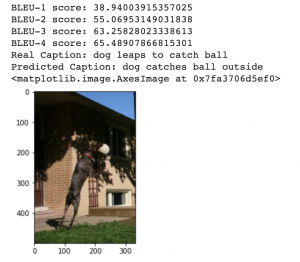```rid = np.random.randint(0, len(img_name_val))
image = img_name_val[rid]
real_caption = ' '.join([tokenizer.index_word[i] for i in cap_val[rid] if i not in ])
caption,result,attention_weights = evaluate(image)

first = real_caption.split(' ', 1)
real_caption = first.rsplit(' ', 1)

for i in caption:
if i=="<unk>":
caption.remove(i)

for i in real_caption:
if i=="<unk>":
real_caption.remove(i)

result_join = ' '.join(caption)
result_final = result_join.rsplit(' ', 1)
real_appn = []
real_appn.append(real_caption.split())
reference = real_appn
candidate = caption

score = sentence_bleu(reference, candidate, weights=(1.0,0,0,0))
print(f"BLEU-1 score: {score*100}")
score = sentence_bleu(reference, candidate, weights=(0.5,0.5,0,0))
print(f"BLEU-2 score: {score*100}")
score = sentence_bleu(reference, candidate, weights=(0.3,0.3,0.3,0))
print(f"BLEU-3 score: {score*100}")
score = sentence_bleu(reference, candidate, weights=(0.25,0.25,0.25,0.25))
print(f"BLEU-4 score: {score*100}")
print ('Real Caption:', real_caption)
print ('Predicted Caption:', ' '.join(caption))
temp_image = np.array(Image.open(image))
plt.imshow(temp_image)
```

Output: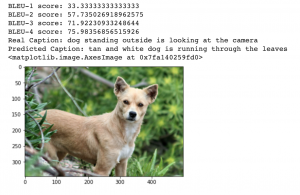#### Step 11:- Comparison

Let’s compare the BLEU scores achieved in the previous article using Bahdanau’s Attention vs our Transformers.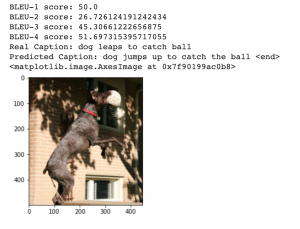The BLEU scores on the left are using Bahdanau’s Attention and the BLEU Scores on the right is using Transformers. As we can see Transformer performs far better than just an attention model.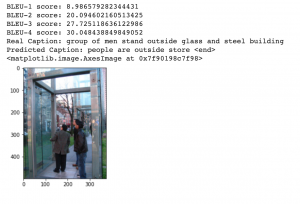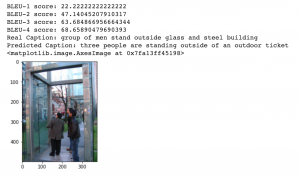And there it is!  We have successfully implemented Transformers using Tensorflow and seen how it can produce a state of the art results.

## End Notes

To summarise, Transformers are better than all the other architectures that we have seen before because they totally avoid recursion, by processing sentences as a whole and by learning relationships between words thanks to multi-head attention mechanisms and positional embeddings. It must also be pointed out that transformers using Tensorflow can capture only dependencies within the fixed input size used to train them.

There are many new powerful transformers like Transformer-XL, Entangled Transformer, Meshed Memory Transformer that can also be implemented for applications like Image Captioning to achieve even better results.